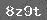# 小学数学第三册第三单元试卷

1. 3×4=(　　　 )，读作(　　　 )乘以(　　　 )等于(　　　 )．表示(　　　 )(　　　 )连加．

2. 写出乘法算式，计算出结果：

52相加：______________

3. 把口诀补充完整．

(　　　 )二得二　　(　　　 )十二　　　(　　　 )十六　　 三三(　　　 )　　 (　　　 )得四

(　　　 )得一　 (　　　 )得八　　　(　　　 )四十二　　 二三(　　　 )　　 (　　　 )得四

4.

○○　 ○○　　 ○○　　 ○○

1.　2×2=

3×4=

4×4=

2×3=

2-2=

2+2=

4×3+4=

4×4-2=

2. 　3+3=

3×3=

3-3=

2×3=

4×1=

17-9=

3×2+3=

4×1+6=

3.　2×4=

20-9=

3×5=

18+7=

5×5=

28+8=

3+3+2=

4×1+8=

1. 被乘数是4，乘数是3____________

2. 写出乘法算式，计算出结果．

34连加：______________

1. 看图列式计算：

ΔΔΔ加法算式：_____________

ΔΔΔ

ΔΔΔ

ΔΔΔ乘法算式：_____________

2. 看图列式计算：

ΔΔ　 ΔΔ

ΔΔ　 ΔΔ

3. 看图列式计算．1. 根据下面的算式画图．

3×5____________

5×3____________

2. 根据下面的算式画图．

(1)2×4

(2)4×2

3. 把左右两边意思一样的算式用线连起来．

(1)2+2+2+2　　　　　　A 5×3

(2)4+4+4　　　　　　　B 3×5

(3)5+5+5　　　　　　　C 2×4

(4)3+3+3+3+3　　　　　D 4×3

### 相关推荐

 留言与评论（共有 0 条评论）

 验证码：